# Equation of a line in two intercept form

Coordinate geometry

Straight Lines: Two intercept form

We need at least two points to determine the nature of a line. This analogy is used to quickly sketch a line if its equation is given in the Two Intercept-Form. Consider the following graph where a line L makes x-intercept at a and y-intercept at b on the axes. This means that L meets x-axis at the point (a, 0) and y-axis at the point (0, b).Use the two-point form of the equation to substitute the values of the intercepts:Again, a= x-intercept and b= y-intercept

To convert the equation into a slope-intercept form may y as the subject:Example 1. Determine the equation of the line with x-intercept 4 and y-intercept 3.

x-intercept a = 4.

y-intercept b = 9.

Substitute in the formula asExample 2. Find the equation of a straight line with X-intercept A(-7,0) and Y-intercept B(0,14).

From the above information, we know the X-intercept is a=-7 and the Y-intercept is b=14. Now we substitute the values in the formula of the intercepts form as given:Example 3. Convert general form of the equation into intercept form for 9x-5y=4Example 4. Reduce the general equation -8x+5y = 9 into intercept form and find the x-intercept and y-intercept.From the equation above, the x-intercept is -9/8 and y-intercept is 9/5.

Example 5. Find the x- and y-intercepts of the line using these coordinates, (–4, –5) and (6, 2).

Convert the points to intercept form equation by:

 Using slope-intercept form Using two-point form y = mx + b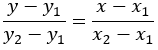Find m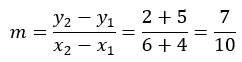Substitute the values of an ordered pair to form general equation: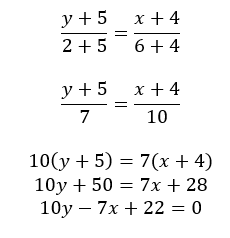Substitute (6, 2 ) in the slope-intercept form to obtain y-intercept: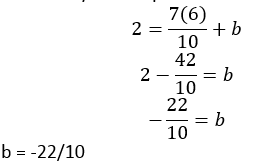Convert the general equation into intercept form: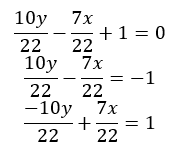Substitute m and b to find x intercept when y=0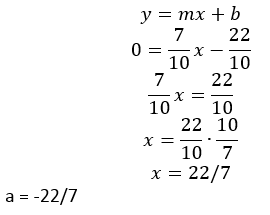Shift the coefficient of x and y to the denominators: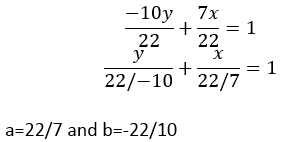Substitute the values of a and b in the intercept form: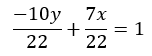The equation is written as: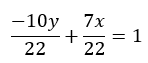Posted in A2